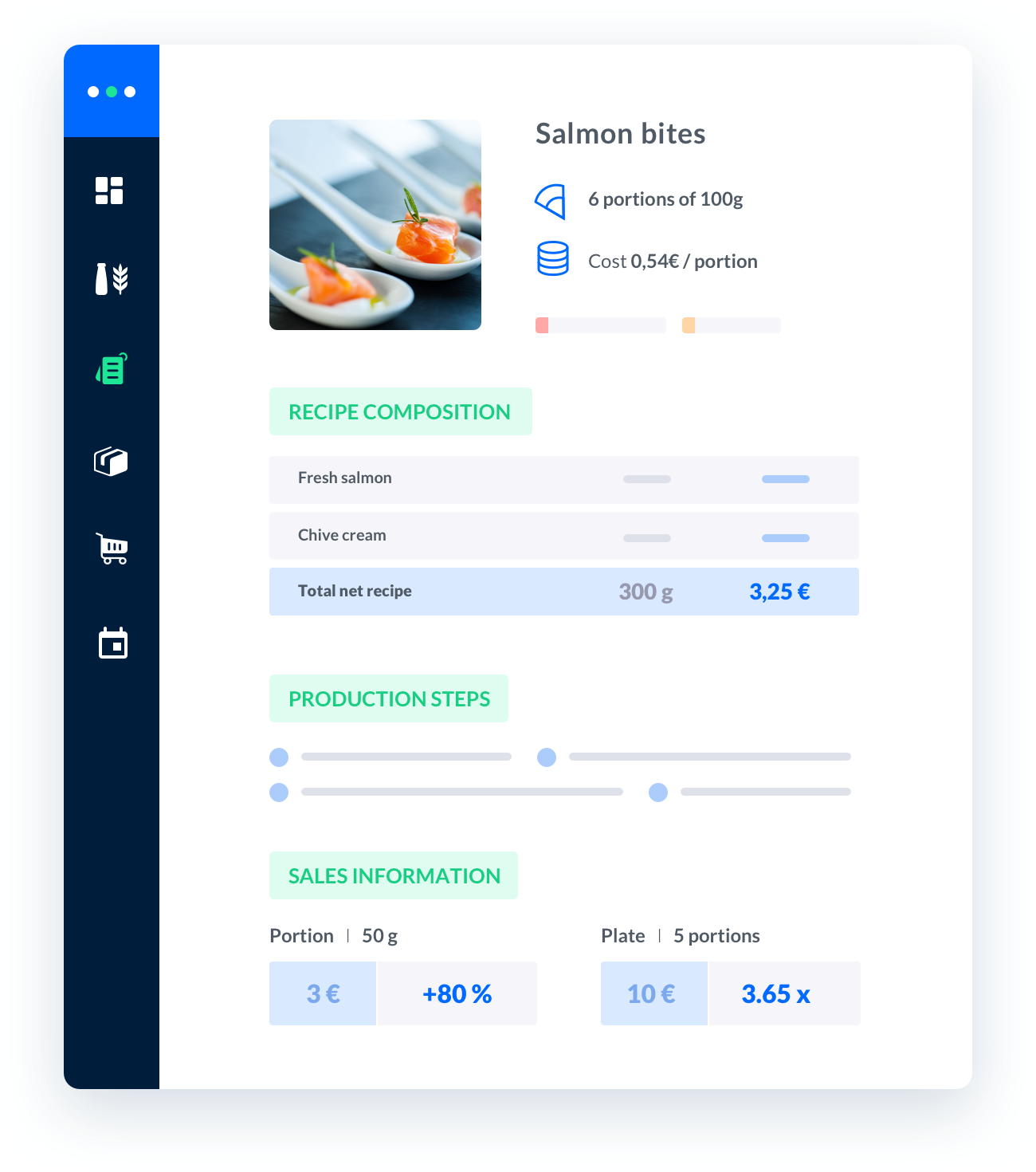# food cost formula pdf

In the catering industry, it is crucial to have a solid understanding of food cost formulas.Calculating food costs accurately is essential for managing profitability and ensuring the success of your business.

Published on 01/01/2023Sébastien Vassaux##### Melba: the food cost app to optimize the profitability of your restaurant

Discover how to optimize the profitability of your restaurant with melba##### The ultimate guide to food cost restaurant

In the catering industry, it is crucial to have a solid understanding of food cost formulas. Calculating food costs accurately is essential for managing profitability and ensuring the success of your business. In this article, we will explore the food cost formula and provide a comprehensive guide on how to calculate it effectively. You can also find a downloadable PDF version of the formula at the end of this article.

## The Importance of Food Cost Formula

Understanding and utilizing the food cost formula is vital for any professional in the catering industry. It allows you to determine the cost of individual menu items accurately, taking into account the cost of ingredients, labor, overheads, and other expenses. By calculating food costs, you can assess the profitability of your menu items and make informed decisions to maximize your revenue.

### 1. Calculating the Cost of Ingredients

When calculating food costs, the first step is to determine the cost of ingredients. This includes all the items used to prepare a particular dish. To calculate the ingredient cost, you need to consider the purchase price, the quantity used, and any waste or trimmings.

For example, let's say you are preparing a pasta dish that requires 200 grams of pasta, 100 grams of sauce, and 50 grams of cheese. If the cost of pasta is \$2 per kilogram, the cost of sauce is \$3 per kilogram, and the cost of cheese is \$5 per kilogram, the ingredient cost for this dish would be:

• Pasta: 200 grams * \$2 per kilogram = \$0.40
• Sauce: 100 grams * \$3 per kilogram = \$0.30
• Cheese: 50 grams * \$5 per kilogram = \$0.25

Therefore, the total ingredient cost for this dish would be \$0.40 + \$0.30 + \$0.25 = \$0.95.

### 2. Calculating Labor Costs

In addition to the cost of ingredients, it is essential to consider the labor costs associated with preparing a particular dish. Labor costs include the wages of the kitchen staff involved in the preparation process. To calculate labor costs, you need to determine the number of hours spent on preparing the dish and the hourly wage for each staff member.

For instance, if it takes a cook 30 minutes to prepare the pasta dish mentioned earlier, and the cook's hourly wage is \$15, the labor cost for this dish would be:

• 30 minutes / 60 minutes per hour = 0.5 hours
• 0.5 hours * \$15 per hour = \$7.50

Therefore, the labor cost for this dish would be \$7.50.

Besides ingredient and labor costs, overhead costs play a significant role in determining the overall food cost. Overhead costs include expenses such as rent, utilities, insurance, and other operational costs. To calculate the overhead cost, you need to determine the percentage of these costs allocated to your menu items.

For example, if your monthly overhead costs amount to \$10,000 and you estimate that 20% of these costs can be attributed to the pasta dish, the overhead cost for this dish would be:

• \$10,000 * 0.20 = \$2,000

Therefore, the overhead cost for this dish would be \$2,000.

## Calculating the Food Cost Percentage

Now that we have determined the ingredient, labor, and overhead costs for a specific dish, we can calculate the food cost percentage. The food cost percentage represents the percentage of the selling price that goes towards covering the cost of producing the dish.

To calculate the food cost percentage, you need to divide the total cost of producing the dish by the selling price, and then multiply the result by 100.

For example, if the selling price of the pasta dish is \$10, the total cost of producing the dish (including ingredients, labor, and overheads) is \$0.95 + \$7.50 + \$2,000 = \$2,008.45. The food cost percentage for this dish would be:

• (\$2,008.45 / \$10) * 100 = 2008.45%

Therefore, the food cost percentage for this dish would be approximately 2008.45%.

## Conclusion

Calculating food costs using the food cost formula is crucial for managing profitability in the catering industry. By accurately assessing the cost of ingredients, labor, and overheads, you can determine the food cost percentage and make informed decisions to maximize your revenue. Remember to review and adjust your food costs regularly to ensure your menu items are priced appropriately for profitability.